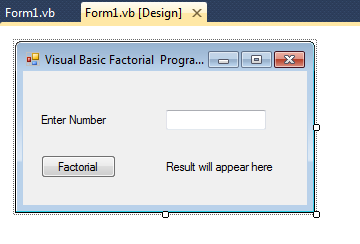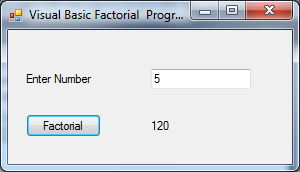# Visual Basic - Factorial of a Number using Recursive Function

I have given here VB Progarm to find a factorial of a number using recursion.

It is designed using WinForm. Winform would look like,The source code to find factorial is given below:

```    Public Class Form1

Private Function Fact(ByVal n As Integer)

If (n = 0) Then
Fact = 1
Else
Fact = n * Fact(n - 1)
End If

End Function

Private Sub Button1_Click(ByVal sender As System.Object, ByVal e As System.EventArgs) Handles Button1.Click

Dim n As Integer
n = Integer.Parse(TextBox1.Text)
Label2.Text = Fact(n).ToString()

End Sub
End Class

```

The output screen would be,